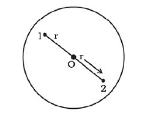Deepak Scored 45->99%ile with Bounce Back Crack Course. You can do it too!

# Consider a sphere of radius R with charge density distributed as

Question:

Consider a sphere of radius R with charge density distributed as

ρ (r) = kr for ≤ R

= 0 for r > .

(a) Find the electric field at all points r.

(b) Suppose the total charge on the sphere is 2e where e is the electron charge. Where can two protons be embedded such that the force on each of them is zero. Assume that the introduction of the proton does not alter the negative charge distribution.

Solution:

(a)

The symmetry of the problem suggests that the electric field is radial.

For points $r (a) Consider Gaussian surfaces as shown in figure given below. For points at$r

$\oint \overrightarrow{\mathrm{E}} \cdot \overrightarrow{\mathrm{dS}}=\frac{1}{\varepsilon_{0}} \int p d V$

Now, $V=\frac{4}{3} \pi r^{3} \Rightarrow d V=3 \times \frac{4}{3} \pi r^{3} d r$ or $d V=4 \pi r^{2} d r$

$\Rightarrow \oint \mathrm{E} \cdot \mathrm{dS}=\frac{1}{\varepsilon_{0}} 4 \pi K \int_{0}^{r} r^{3} d r[\because p(r)=K r]$

$\Rightarrow(E) 4 \pi r^{2}=\frac{4 \pi K}{\varepsilon_{0}} \frac{r^{4}}{4}$

$\Rightarrow E=\frac{1}{4 \varepsilon_{0}} \mathrm{Kr}^{2}$

Here, charge density is positive. Therefore, direction of $\mathbf{E}$ is radially outwards.

For points $r>R$,

$\oint \mathrm{E} \cdot \mathrm{dS}=\frac{1}{\varepsilon_{0}} \int p \cdot d V$

$\Rightarrow E\left(4 \pi r^{2}\right)=\frac{4 \pi K}{\varepsilon_{0}} \int_{0}^{R} r^{3} d r=\frac{4 \pi K}{\varepsilon_{0}} \frac{R^{4}}{4}$

$\Rightarrow E=\frac{K}{4 \varepsilon_{0}} \frac{R^{4}}{r^{2}}$

Now charge density is again positive. So, the direction of $E$ is radially outward.

(b)

The two protons must be on the opposite sides of the centre along a diameter. Suppose the protons are at a distance $r$ from the centre.$q=\int_{0}^{\pi} p d V=\int_{0}^{8}(K r) 4 \pi r^{2} d r$

$q=4 \pi K \frac{R^{4}}{4}=2 e$

$\therefore k=\frac{2 e}{\pi R^{4}}$

Consider the forces on proton 1 . The attractive force due to the charge distribution is

$F_{1}=-e E=\frac{-e K r^{2}}{4 \varepsilon_{0}}$

Repulsive force on proton 1 due to proton 2 is

$F_{2}=\frac{e^{2^{2}}}{4 \pi \varepsilon_{0}(2 r)^{2}}$

So, $F_{N n}=\left[\frac{-e r^{2}}{4 \varepsilon_{0}} \frac{Z e}{\pi R^{4}}+\frac{e^{2}}{16 \pi \varepsilon_{0} r^{4}}\right]$

Thus, net force on proton 1 will be zero. when

$\frac{e r^{2} 2^{*}}{4 \varepsilon_{0} \pi R^{4}}=\frac{e^{2}}{16 \pi \varepsilon_{0} r}$

$\Rightarrow r^{4}=\frac{R^{4}}{8}$

$\Rightarrow r=\frac{R}{(8)^{1 / 4}}$

Therefore, distance of both the protons from the centre must be $r=\frac{R}{(8)^{1 / 4}}$.